# [Solved] Use the One-To-One Property to Solve the Following Equation for X

Question 252
Multiple Choice

## Use the One-to-One Property to solve the following equation for x.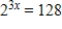​

A)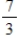B)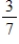C)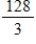D)2
E)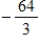10+ million students use Quizplus to study and prepare for their homework, quizzes and exams through 20m+ questions in 300k quizzes.

### Mathematics

Explore our library and get Calculus Homework Help with various study sets and a huge amount of quizzes and questions

137

Study sets

2K

Quizzes

233.3K

Questions

Upload material to get free accessInvite a friend and get free accessSubscribe and get an instant access